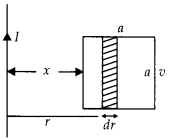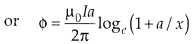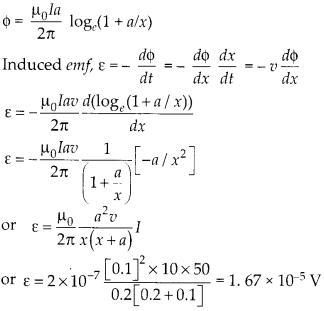Enlightened

# Question 16: NCERT Solutions for 12th Class Physics: Chapter 6-Electromagnetic Induction

• 0

Question 16: NCERT Solutions for 12th Class Physics: Chapter 6-Electromagnetic Induction

(a) Obtain an expression for mutualin ductance , between a long straight wire and a square loop of side ‘a’ as shown in figure.(b) Now assume that straight wire carries a current of 50 A and the loop is moved to the right with a constant velocity, v = 10 m s-1. Calculate the induced emf in the loop at the instant when x = 0.2 m. Take a = 0.1m and assume that loop has a large resistance.

Share

1. Solution:
(a) As the magnetic field will be variable with distance from long straight wire, so the flux through square loop can be calculated by integration.Let us assume a width ‘dr’ of the square loop at a distance ‘r’ from straight wire(b) The square loop is moving right with a constant speed v, the instantaneous flux can be taken asCheck the complete chapter with solutions.

NCERT Solutions for 12th Class Physics: Chapter 6-Electromagnetic Induction

• 0